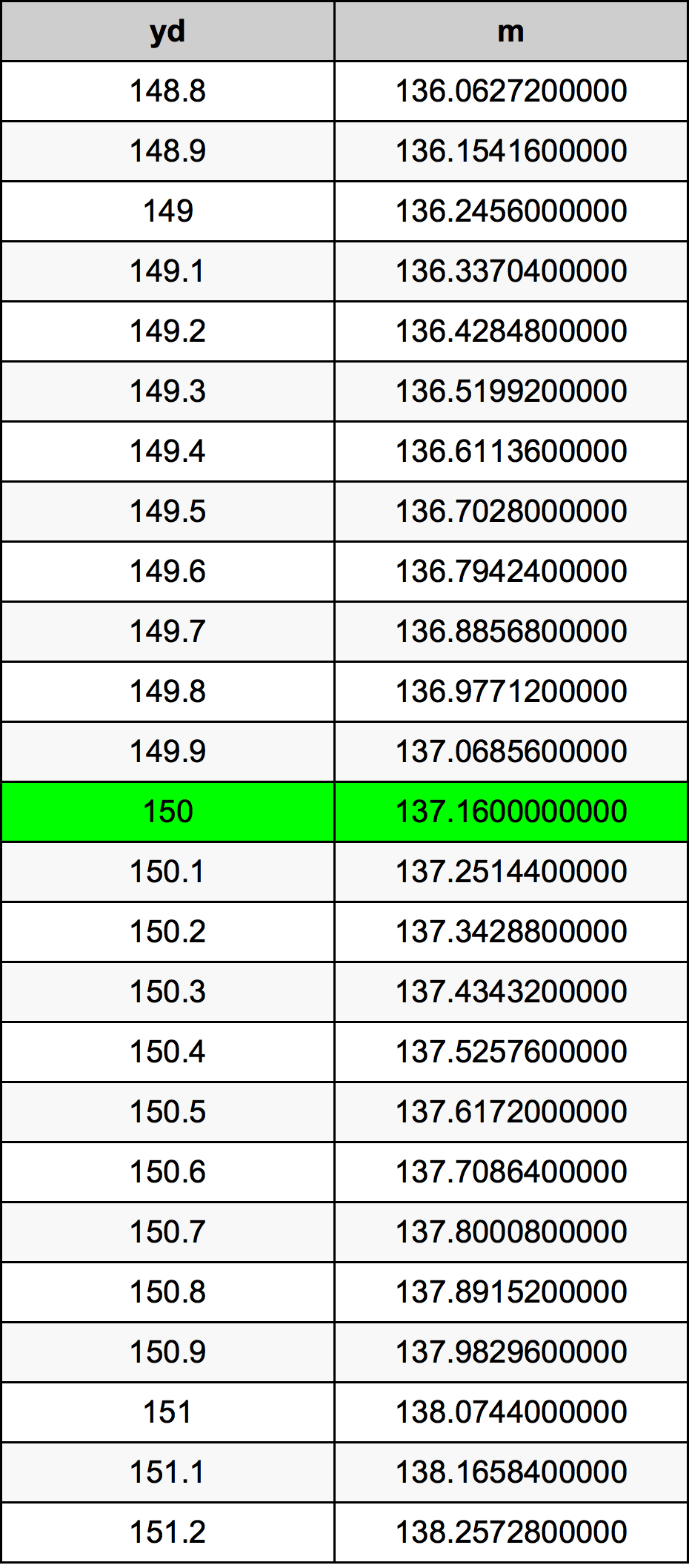Yards To Meters

# 150 yd to m150 Yards to Meters

yd
=
m

## How to convert 150 yards to meters?

 150 yd * 0.9144 m = 137.16 m 1 yd
A common question is How many yard in 150 meter? And the answer is 164.041994751 yd in 150 m. Likewise the question how many meter in 150 yard has the answer of 137.16 m in 150 yd.

## How much are 150 yards in meters?

150 yards equal 137.16 meters (150yd = 137.16m). Converting 150 yd to m is easy. Simply use our calculator above, or apply the formula to change the length 150 yd to m.

## Convert 150 yd to common lengths

UnitLengths
Nanometer1.3716e+11 nm
Micrometer137160000.0 µm
Millimeter137160.0 mm
Centimeter13716.0 cm
Inch5400.0 in
Foot450.0 ft
Yard150.0 yd
Meter137.16 m
Kilometer0.13716 km
Mile0.0852272727 mi
Nautical mile0.0740604752 nmi

## What is 150 yards in m?

To convert 150 yd to m multiply the length in yards by 0.9144. The 150 yd in m formula is [m] = 150 * 0.9144. Thus, for 150 yards in meter we get 137.16 m.

## 150 Yard Conversion Table## Alternative spelling

150 Yards to Meters, 150 Yards in Meters, 150 Yard to Meter, 150 Yard in Meter, 150 Yards to Meter, 150 Yards in Meter, 150 yd to m, 150 yd in m, 150 Yard to m, 150 Yard in m, 150 yd to Meters, 150 yd in Meters, 150 yd to Meter, 150 yd in Meter